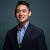# Graph/Dynamic Programming/Heap — Cheapest Flights Within K Stops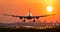https://www.savethestudent.org/travel/how-to-get-cheap-flights-as-a-student.html

There are `n` cities connected by `m` flights. Each flight starts from city `u` and arrives at `v` with a price `w`.

Now given all the cities and flights, together with starting city `src` and the destination `dst`, your task is to find the cheapest price from `src` to `dst` with up to `k` stops. If there is no such route, output `-1`.

`Example 1:Input: n = 3, edges = [[0,1,100],[1,2,100],[0,2,500]]src = 0, dst = 2, k = 1Output: 200Explanation: The graph looks like this:`

# Dynamic Programming — Coin Change 2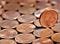Reference: https://www.themayor.eu/ro/france-could-soon-remove-1-and-2-eurocent-coins

Given coins of different denominations and a total amount of money. Write a function to compute the number of combinations that make up that amount, assuming that you have infinite number of each kind of coin.

`Input: amount = 5, coins = [1, 2, 5]Output: 4Explanation: there are four ways to make up the amount:5=55=2+2+15=2+1+1+15=1+1+1+1+1`

This is another classic dynamic programming problem where it can be solved with a more straightforward recursive approach and then optimized with iterative approach.

# Solution 1:

`State: 1. Parameters: total - current amount, start - index of the input…`

# Dynamic Programming — Minimum Cost to Reach the End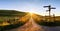Reference: https://www.sjpl.org/social-work

Given an array of integers and integer K, each integer in the array represents the cost to reach the corresponding index and K represents the maximum jump one can take. The goal is to find the minimum cost to reach the last index in the array starting from the beginning.

`Input:  arr = [20, 30, 40, 25, 15, 20, 28], K = 3Output:   73Explanation: Since K = 3, we can start from 30, and then take 3 jumps to 15, and lastly 28 (30 + 15 + 28). Alternatively, start from 20, take 3 jumps to 25, and…`

# Graph — Alien Dictionary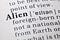Reference: https://www.dreamstime.com/definition-alien-fake-dictionary-word-image135385630

There is a new alien language which uses the latin alphabet. However, the order among letters are unknown to you. You receive a list of non-empty words from the dictionary, where words are sorted lexicographically by the rules of this new language. Derive the order of letters in this language.

`Input:[  "wrt",  "wrf",  "er",  "ett",  "rftt"]Output: "wertf"Input:[  "z",  "x"]Output: "zx"Input:[  "z",  "x",  "z"] Output: "" Explanation: The order is invalid, so return "".`

# Solution:

`Intuition: Build a graph w each character as a vertex and dependency as an edge…`

# Heap — Merge k Sorted Lists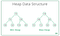Reference: https://www.geeksforgeeks.org/heap-data-structure/

Merge k sorted linked lists and return it as one sorted list.

`Input:[  1 -> 4 -> 5,  1 -> 3 -> 4,  2 -> 6]Output: 1 -> 1 -> 2 -> 3 -> 4 -> 4 -> 5 -> 6`

# Solution:

`Intuition: Having the head node of each sorted linked lists, we can implement a min heap that first push all the head nodes onto the min heap. Then pop the smallest one and push its next node onto the min heap in each iteration. The loop will terminate when the heap queue is empty.…`

# String Manipulation — Minimum Window Substring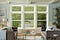Reference: https://www.windowworldhouston.com/windows/double-hung/

Given a string S and a string T, find the minimum window in S which will contain all the characters in T in complexity O(n).

`Input: s = "ADOBECODEBANC", t = "ABC"Output: "BANC"Input: s = "ABCDEFGF", t = "HIJKL"Output: ""Input: s = "ABCDEFGF", t = "DEFF"Output: "DEFGF"Input: s = "b", t = "bb"Output: ""Input: s = "abc, t = "b"Output: "b"`

# Solution 1:

`Intuition: Use nested while loops to find the minimum sub string that starts from each character and return the smallest one.`
1. Use the collections library’s counter to create…

# String Manipulation — Perform String Shifts

You are given a string `s` containing lowercase English letters, and a matrix `shift`, where `shift[i] = [direction, amount]`

0 means left shift, and 1 means right shift.

`Input: s = "abc", shift = [[0, 1], [1, 2]]Output: "cab"`

# Solution:

`Shifting the string as we traverse through the shift array would be too expensive. Instead, we can just count the total left shift times and shift the string once.`
1. For loop iterate through the shift array
2. Inside the for loop, add if it’s left shift, subtract if it’s right shift.
3. If the number of total left shifts needed…

# Binary Search — Find K-th Smallest Pair Distance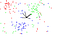Reference: https://www.unite.ai/what-is-k-nearest-neighbors/

Given an integer array, return the k-th smallest distance among all the pairs. The distance of a pair (A, B) is defined as the absolute difference between A and B.

`Let nums be the input array, k be the targeted pair, and n be the size of the input arraynums = [1, 3, 1], k = 2 -> d = 2Explanation: (1, 1), (1, 3), (1, 3) or (3, 1)nums = [0, 0, 0, 0, 1, 1, 1, 2, 2, 2], k = 5 -> d = 0 Explanation: Of all the 45 pairs, know…`

# Graph — Number of Islands II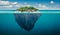Reference: https://gist.github.com/huang983/71efca0f49ca3bf4fd278f0ab2f6abb9

Given a water 2D matrix and an array of positions that need to be turned to lands, find the number of islands after each operation

positions = [[0, 0]. [1, 1], [2, 2], [0, 2], [2, 0]]

`water matrix:       0 0 0 0 0 0 0 0 0[0, 0]:   1 0 0 0 0 0 0 0 0 1 island[1, 1]:  1 0 00 1 0 0 0 0 2 islands[2, 2]: 1 0 0 0 1 0 0 0 1 3 islands[0, 2]: 1 0 1  0 1 0 0 0 1 …`